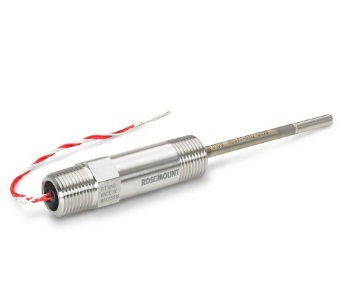# WHAT IS RESISTANCE THERMOMETERS ?## Introduction

Resistance thermometers is also called RTD (resistance temperature detector). It operates on the principle that, electrical resistance of a substance changes with temperature. The substance may be a semiconductor metal. The traditional sensing element of a resistance thermometer consists of a coil of small wire diameter to a precise resistance value. The most common material is platinum, although nickel, copper, and nickel-iron alloys compete with platinum in many applications.

## Principle

Resistance of most metals increases with temperature. The variation of electrical resistance with temperature may be expressed as:
R = (R0 + aT)
Where R is the resistance, R0 is the resistance at 0 ºC , T is the temperature and a is a constant. This is a generalization and cannot be used in resistance thermometry as a linear relationship of resistance and temperature is assumed over only a small temperature range. The resistance temperature relation for platinum is given by Callendar equation.

T = [(Rt-R0)/(R100-R0)]100 +d[(T/100)-1] (T/100)

Where T is the temperature at resistance element, Rt is the resistance at temperature T, R0 is the resistance at 0 ºC , R100 is the resistance at 100 degree C and d is a constant, characteristic of each element.

## RTD Materials

The material must have

(i) a continuous and stable relationship between resistance and temperature and

(ii) high thermal coefficient of resistance.

Platinum is a noble metal and has the most stable temperature resistance ratio in the largest temperature range. Nickel elements have a limited temperature range because the amount of change in resistance per degree of change in temperature becomes very non-linear at temperatures above 572 ° F (300 ° C). Copper has a very linear temperature resistance ratio, however, copper oxidizes at moderate temperatures and can not be used at more than 302ºF (150ºC).Platinum is the best metal for RTD because it follows a very linear temperature resistance relationship and follows the  R vs. T ratio in a highly repeatable way over a wide temperature range. The unique properties of platinum make it the material of choice for temperature standards in the range of -272.5 ° C to 961.78 ° C, and is used in the sensors that define the International Standard for Temperature, ITS-90 between -190 ° C  and 660ºC

• 2 wire RTD
• 3 wire RTD
• 4 wire RTD

## Construction

The industrial resistance thermometer is a combination of a thermometer bulb, a measuring circuit and an automatic balancing potentiometric instrument. The thermometer bulb is manufactured in various forms and consists of a thin wire coil wound in insulating material. The resistance wire is arranged so that a good thermal conductivity is provided and a high heat transfer rate is obtained. The windings must be made in such a way that the physical tension is negligible as the cable expands and contracts with the change in temperature. The resistance bulb is tempered and aged after manufacture to stabilize its electrical properties. The most common measurement method is the Wheatstone bridge with several modifications necessary for use with a resistance thermometer bulb.1. High accuracy
2. Simplicity
3. Wide operating range
4. Suitable for precision applications
5. Temperature changes as small as 0.030F can be measured without difficulty.

### Limitation

RTDs in industrial applications are rarely used above 660 °C. At temperatures above 660 °C it becomes increasingly difficult to prevent the platinum from becoming contaminated by impurities from the metal sheath of the thermometer.